A tube of length L is filled completely with an incompressible liquid of mass M and closed at both ends. The tube is then rotated in a horizontal plane about one of its ends with a uniform angular velocity $\omega$. The force exerted by liquid at the other end is

1.  $\frac{{\mathrm{M\omega }}^{2}\mathrm{L}}{2}$

2.  ${\mathrm{ML\omega }}^{2}$

3.  $\frac{{\mathrm{M\omega }}^{2}\mathrm{L}}{4}$

4.  $\frac{M{L}^{2}{\omega }^{2}}{2}$

Concept Questions :-

Pressure
NEET 2021 - Achiever Batch - Aryan Raj Singh

Difficulty Level:

The radius of a soap bubble is increased from R to 2 R. Work done in this process (T = surface tension) is

1.

2.

3.

4.

Concept Questions :-

Surface tension
NEET 2021 - Achiever Batch - Aryan Raj Singh

Difficulty Level:

A small spherical solid ball is dropped in a viscous liquid. Its journey in the liquid is best described in the figure by :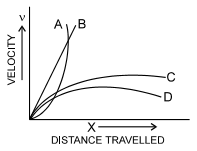1.  Curve A

2.  Curve B

3.  Curve C

4.  Curve D

Concept Questions :-

Stokes' law
NEET 2021 - Achiever Batch - Aryan Raj Singh

Difficulty Level:

Water flows through a frictionless duct with a cross-section varying as shown in the figure. Pressure p at points along the axis is represented by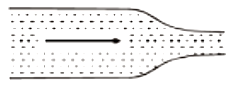1.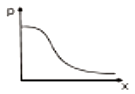2.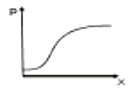3.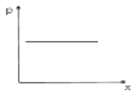4.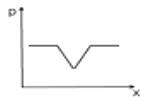Concept Questions :-

Equation of continuity
NEET 2021 - Achiever Batch - Aryan Raj Singh

Difficulty Level:

The area of cross-section of the wider tube shown in the figure is  If a mass of 12 kg is placed on the massless piston, the difference is heights h in the level of water in the two tubes is :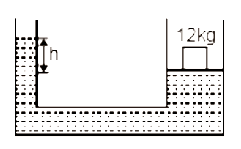1.  10 cm

2.  6 cm

3.  15 cm

4.  2 cm

Concept Questions :-

Properties of fluids
NEET 2021 - Achiever Batch - Aryan Raj Singh

Difficulty Level:

Equation of continuity based on :

1.  Conservation of mass

2.  Conservation of energy

3.  Conservation of angular momentum

4.  None of these

Concept Questions :-

Equation of continuity
NEET 2021 - Achiever Batch - Aryan Raj Singh

Difficulty Level:

The weight of an aeroplane flying in the air is balanced by

(1) Vertical component of the thrust created by air currents striking the lower surface of the wings

(2) Force due to reaction of gases ejected by the revolving propeller

(3) Upthrust of the air which will be equal to the weight of the air having the same volume as the plane

(4) Force due to the pressure difference between the upper and lower surfaces of the wings created by different air speeds on the surfaces

Concept Questions :-

Bernoulli theorem
NEET 2021 - Achiever Batch - Aryan Raj Singh

Difficulty Level:

A U tube with both ends open to the atmosphere,is partially filled with water. Oil, which is immiscible with water, is poured into one side until it stands at a distance of 10mm above the water level on the other side. Meanwhile the water rises by 65 mm from its original level (see diagram). The density of the oil is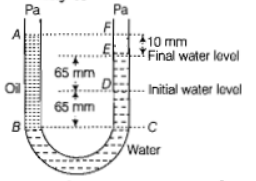(a)

(b)

(c)

(d)

Concept Questions :-

Properties of fluids
NEET 2021 - Achiever Batch - Aryan Raj Singh

Difficulty Level:

A rectangular film of liquid is extended from (4 cm) to . If the work done is $3×{10}^{-4}$ J,the value of the surface tension of the liquid is

(a)

(b)

(c) $0.2N{m}^{-1}$

(d)

Concept Questions :-

Surface tension
NEET 2021 - Achiever Batch - Aryan Raj Singh

Difficulty Level:

Three liquids of densities  (with ${\rho }_{1}>{\rho }_{2}>{\rho }_{3}$), having the same value of surface tension T, rise to the same height in three identical capillaries. The angles of contact  obey

(a) $\frac{\pi }{2}>{\theta }_{1}>{\theta }_{2}>{\theta }_{3}\ge 0$

(b) $0\le {\theta }_{1}<{\theta }_{2}<{\theta }_{3}<\frac{\pi }{2}$

(c) $\frac{\pi }{2}<{\theta }_{1}<{\theta }_{2}<{\theta }_{3}<\pi$

(d) $\pi >{\theta }_{1}>{\theta }_{2}>{\theta }_{3}>\frac{\pi }{2}$

Concept Questions :-

Surface tension